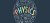# Computational Physics

Free!

language English Optional, paid
Category:

This course aims to give the students competence in the methods and techniques of calculations using computers. At the end of the course the student is expected to have a hands on experience in modeling, algorithm development, implementation and calculation of physical quantities of relevance in interacting many body problems in physics. Both quantum and classical computational tools will be introduced.

COURSE LAYOUT

Week 1: Rapid overview of Fortran programming Language
Week 2: Random Number generation and testing, Generation of random numbers with given distribution
Week 3: Numerical Integration: (a) Deterministic: Trapezoidal method & (b) Multi-dimensional Integration
using stochastic methods.
Week 4: Lattice Monte Carlo simulations using Ising model to understand phase transitions: Metropolis
algorithm, understanding kinetic barriers, finite size effects, role of thermal fluctuations
Week 5: Metropolis algorithm, understanding kinetic barriers, finite size effects, role of thermal fluctuations;
Principle of detailed balance, calculating thermodynamic averages
Week 6: Determining transition temperature using Binders cumulant
Week 7: Solving differential equations
Week 8: Linear, non-linear and coupled differential equations
Week 9: Solving differential equations Schrodinger eqn. in Quantum Mechanics with Numerov’s algorithm
and variational principle.
Week 10: Classical Molecular Dynamics simulations using Lennard-Jones’ potential
Week 11: Classical Molecular Dynamics simulations using Lennard-Jones’ potential
Week 12: Classical Molecular Dynamics simulations using Lennard-Jones’ potential.

## User Reviews

0.0 out of 5
0
0
0
0
0

There are no reviews yet.

×